Hajdarevic, S., Obucina, M., Mesic, E., and Martinovic, S. (2020). "Strength and stiffness analyses of standard and double mortise and tenon joints," BioRes. 15(4), 8249-8267.

#### Abstract

This paper investigated the effect of the tenon length on the strength and stiffness of the standard mortise and tenon joints, as well of the double mortise and tenon joints, that were bonded by poly(vinyl acetate) (PVAc) and polyurethane (PU) glues. The strength was analyzed by measuring applied load and by calculating ultimate bending moment and bending moment at the proportional limit. Stiffness was evaluated by measuring displacement and by calculating the ratio of applied force and displacement along the force line. The results were compared with the data obtained by the simplified static expressions and numerical calculation of the orthotropic linear-elastic model. The results indicated that increasing tenon length increased the maximal moment and proportional moment of the both investigated joints types. The analytically calculated moments were increased more than the experimental values for both joint types, and they had generally lower values than the proportional moments for the standard tenon joints, as opposed to the double tenon joints. The Von Mises stress distribution showed characteristic zones of the maximum and increased stress values. These likewise were monitored in analytical calculations. The procedures could be successfully used to achieve approximate data of properties of loaded joints.

Strength and Stiffness Analyses of Standard and Double Mortise and Tenon Joints

Seid Hajdarevic,a,* Murco Obucina,a Elmedin Mesic,b and Sandra Martinovic a

This paper investigated the effect of the tenon length on the strength and stiffness of the standard mortise and tenon joints, as well of the double mortise and tenon joints, that were bonded by poly(vinyl acetate) (PVAc) and polyurethane (PU) glues. The strength was analyzed by measuring applied load and by calculating ultimate bending moment and bending moment at the proportional limit. Stiffness was evaluated by measuring displacement and by calculating the ratio of applied force and displacement along the force line. The results were compared with the data obtained by the simplified static expressions and numerical calculation of the orthotropic linear-elastic model. The results indicated that increasing tenon length increased the maximal moment and proportional moment of the both investigated joints types. The analytically calculated moments were increased more than the experimental values for both joint types, and they had generally lower values than the proportional moments for the standard tenon joints, as opposed to the double tenon joints. The Von Mises stress distribution showed characteristic zones of the maximum and increased stress values. These likewise were monitored in analytical calculations. The procedures could be successfully used to achieve approximate data of properties of loaded joints.

Keywords: Mortise and tenon joint; Strength; Stiffness; Tenon length; Glue; Finite element method

Contact information: a: Department of Wood Technology, Faculty of Mechanical Engineering, University of Sarajevo, 71000, Sarajevo, Bosnia and Herzegovina; b: Department of Mechanical Design, Faculty of Mechanical Engineering, University of Sarajevo, 71000, Sarajevo, Bosnia and Herzegovina;

* Corresponding author: hajdarevic@mef.unsa.ba

INTRODUCTION

Mortise and tenon joints have a wide range of use in furniture frame construction. Although this type of joint is a common and basic way of connecting wooden elements into a frame, there is still a considerable interest in analyzing the existing and developing new forms of the tenon joint. A better understanding of speciﬁc mechanical properties of tenon joints can lead to improvement of quality of furniture frame structure.

There are numerous factors that affect the mechanical properties of loaded profile-adhesive joints. The bending moment capacity of the window joints shows a statistically significant difference between bending moments for the tenon and dowel joints, and the absence of difference between the compression and tension for both joint types (Podlena et al. 2017). The factors that affect the moment capacity, and the main effects and interaction factors affecting the moment capacity for both compression and tension loads of the mortise and tenon joints have been investigated (Kasal et al. 2015). Tenon size (width and length) affects the bending moment capacity, and joint capacity is most affected by tenon length. In addition, shear strength of the wood parallel to grain has a substantial effect, while the adhesives have a measurable effect on the joint capacity.

The mortise and tenon furniture joins became stronger and stiffer as either tenon width or length increased. Tenon length has a greater effect on moment resistance, while tenon width has more effect on stiffness (Wilczyński and Warmbier 2003; Kasal et al. 2016). Mortise and tenon joints with tight-fitting shoulders have greater bending moment capacity than those with loose fitting shoulders; in addition, tenon shoulders substantially decrease rotation factors. Tenon cross-section also has a substantial effect on bending moment capacity. Joints with a round tenon configuration have only half of the capacity values of those with rectangular tenon configuration (Likos et al. 2012). The bending moment capacity is directly related to the depth of embedment of the tenons and strongly related to shoulder width, whereas tenon width have a lesser effect (Derikvand et al. 2014). Analysis of stiffness coefficients of mortise and tenon joints used on wooden window profiles confirmed that type of load did not affect the stiffness of the joint, but the width of joint did affect the stiffness (Podlena and Boruvka 2016). The effect of fitting for pairing of open full-width mortise and tenon joint elements on the compressive strength of the joint has been investigated. The mortise and tenon joints were found to be the strongest at a tight fit of 0.1 mm (Elek et al. 2020).

The results of investigation of the strength of the two most frequent joints in the upholstered furniture frames (mortise and tenon joints and double dowel joints), constructed with two wood species and the use of two adhesives (PVAc and PU) showed that the mortise and tenon joint in combination with PVAc, provided the best strength for all investigated wood species (Vassiliou et al. 2016). In an investigation of effect of wood species and adhesive type on the stiffness of rail to leg mortise and tenon furniture joints, the type of glue was not important for spruce joints, whereas for beech, the stiffness of joints glued with PVAc was significantly higher than with PU adhesive (Záborský et al. 2017). In comparison with PVAc, PU glue has appropriate strength, and, as they slightly expand during the hardening phase, can completely fill and cover the gaps between elements of joint (Hrovatin et al. 2013). Dowel joints glued with PU adhesive showed improved mechanical properties in humid environments (Máchová et al. 2019). The improved bonding properties of PVAc glue and bending and tension strength of the mortise and tenon joints has been achieved by using nano-fillers and its good dispersion provided at the low loadings of nano-fillers to PVAc matrix (Bardak et al. 2017).

Numerical methods, such as the ‘ﬁnite element method’, are applicable and effective for the analysis of orthotropic structures. The numerical results depend on simpliﬁcations and assumptions introduced in the numerical model. Numerical analyses give reasonable estimates of mechanical properties of wood constructions and their joints (Smardzewski 2008; Horman et al. 2010; Hajdarević and Martinović 2014; Hajdarević and Busuladžić 2015; Hu and Guan 2017; Hu et al 2019a, b).

This study investigated the effect of the tenon length on the mechanical properties of mortise tenon joints taken from a manufacturing process with two different joint geometry that were bonded by three glue types. In addition, the objective was to explore the capabilities of analytical and numerical calculation in design optimization of a frame joint. The strength and stiffness of the standard and double mortise and tenon joints were determined, and the results were compared by the simplified static expressions and numerical calculations of the linear-elastic model.

EXPERIMENTAL

Materials and Specimens

The thirty-six (36) corners mortise and tenon joints that were supplied from furniture manufacturers (MS&WOOD, Fojnica, Bosnia and Herzegovina) were analyzed (Fig. 1). All joints were made from beech wood (Fagus sylvatica L.), with round peg shape and mortise and tenon interference fit. The first set of joints contained 18 standard mortise and tenon joints with cross section of elements of 50 × 30 mm (Fig. 1a), while the second set of joints contained 18 double mortise and tenon joints with cross section of elements 50 × 40 mm and overhanging end (Fig. 1b). The joints were constructed by using two different tenon lengths (20 and 30 mm), with 9 replications of each two joint sets. Two types of glue (PVAc and PU) were utilized for assembling the joint specimens. The three joints specimens, within each joint set and tenon length, were bonded with PVAc glue manufactured by AkzoNobel (PVAc1; Akzo Nobel N.V., Amsterdam, Netherlands) and by Kleiberit 303 D3 (PVAc2; Klebchemie, Weingarten, Germany). Three specimens were bonded with Jowat Power – PUR 687.40 glue (PU; Jowat SE, Detmold, Germany). The moisture content (MC) was evaluated in accordance with procedures describe in ISO 13061-1 (2014) after testing. The average MC value was 12.1%.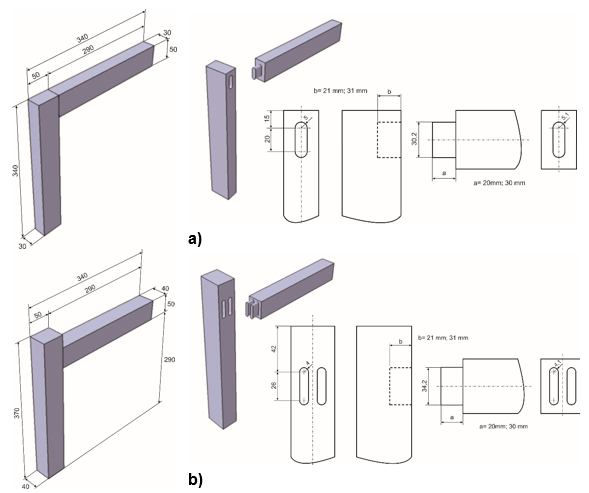Fig. 1. Test samples geometry: a) configuration of the standard mortise and tenon joints with two size of tenon lengths, b) configuration of the double mortise and tenon joints with overhanging end and two size of tenon lengths

Method of Testing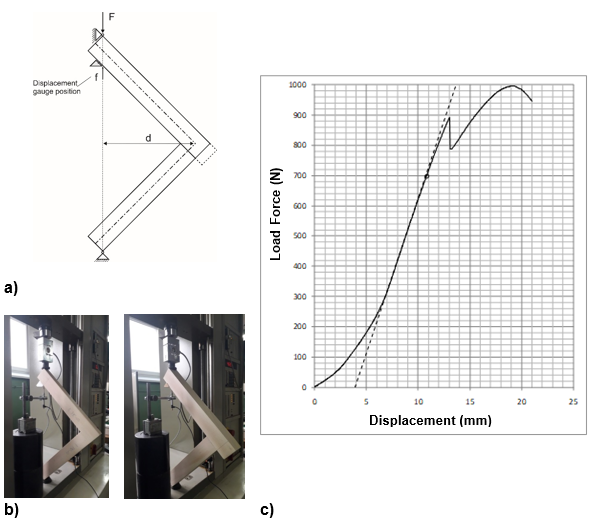Fig. 2. Joints testing: a) the diagram of joint loading, b) set-up of standard and double mortise and tenon joints in the testing machine, c) working diagram of joint strength testing

The ultimate applied load values (Fmax) and the load at the proportional limit (Fp) were ascertained using the software collected data. Corresponding bending moment values of the joints were calculated in Nm by expressions Mmax=Fmax∙d and Mp=Fp∙d for ultimate bending moment and bending moment at the proportional limit, respectively. The moment arm, the perpendicular distance from the force line to the point of intersection of symmetry axis of the elements, was d = 205.06 mm for both set of joints, as shown in Fig. 2a. The values of displacements at the ultimate applied load (δmax) and displacements at the proportional limit (δp) were ascertained using the software collected data and were used for calculation of stiffness that was defined by ratios Fmax/δmax and Fp/δp for ultimate load and load at the proportional limit, respectively. The ratios were calculated in N/mm.

Analytical Calculation of Joints Strength

The strength of mortise and tenon joints was calculated by means of the common simplified static expressions to determine reaction moment of a loaded joint. Figure 3 presents a generalized scheme of analytical consideration of stress distribution on the characteristic support surface of tenon and tenon shoulder along with the required dimensions. The total reaction moment of a joint (1) is the sum of the simultaneous reaction moments obtained on the basis of defined stresses that occur on the characteristic support surfaces of the profile-adhesive joint, Fig. 3a,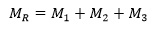(1)

where MR is total reaction moment, M1 is moment of the edge cheek, M2 is moment of the structural shoulder and M3 is moment of the face cheek.

Reaction moments were calculated using the joint dimensions, the number of glue lines and relevant stress (permissible stress or strength of used material), Fig. 3b.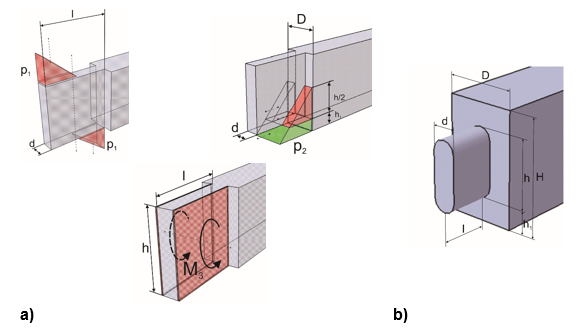Fig. 3. Reaction moments of a loaded joint: a) generalized scheme of stress distribution on the characteristic support surfaces, b) relevant dimensions of standard and double mortise and tenon joints

The moment of the edge cheek M1 and the reaction moment of structural shoulder M2 were obtained by the following equations,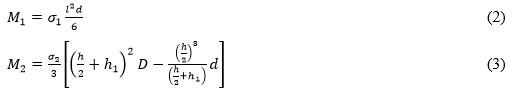where σ1 and σ2 are negative normal stress i.e. compression strength of wood normal to the fibers (Pa), and other notations are shown in Fig. 3b. Moment in the plane of face cheek M3 was obtained by Eq. 3,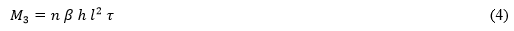where n is the number of face cheeks, τ is shear stress i.e. strength of glue line (Pa), β is the Saint-Venant coefficient shown in Eq. 5,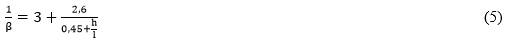where dimensions h and l are tenon width and tenon length.

Numerical Analysis of Joints

The total reactive moments of joints obtained from analytical calculation were used in a simplified numerical analysis of stress and strain of the loaded joints. The physical model is defined based on the experimental loading diagram of the joints and local coordinates are used to define the grain directions of the joint elements, as shown in Fig. 4. Loading forces (Fl) of each model of joints were calculated by the equation FMd where MR is analytical total reaction moment and d is the moment arm.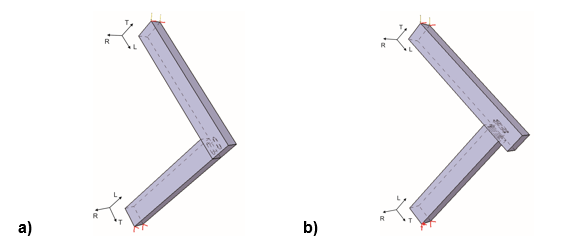Fig. 4. The physical model of the joints: a) standard mortise and tenon joint (tenon length 20 mm), b) double mortise and tenon joint (tenon length 20 mm)

Numerical 3D linear-elastic model for orthotropic material was solved by a method based on the finite elements. Mesh model of standard mortise and tenon joint, along with mesh details of standard and double tenon joints are shown in Fig. 5. The 10-node parabolic tetrahedron finite element was used to create numerical model. The mesh was selectively refined to obtain a better results accuracy. A fastened connection was modeled between surfaces of mortise and tenon while among other surfaces i.e. tenon shoulder and mortise side wall a contact connection was used. Calculation was carried out for beech wood and the elastic properties of the wood are presented in Table 1 (Smardzewski 2008). Adhesive, i.e., glue line and interference fit were neglected. The numerical results were obtained using the CAD/CAM/CAE system CATIA (Dassault Systemes SE, Velizy-Villacoublay, France).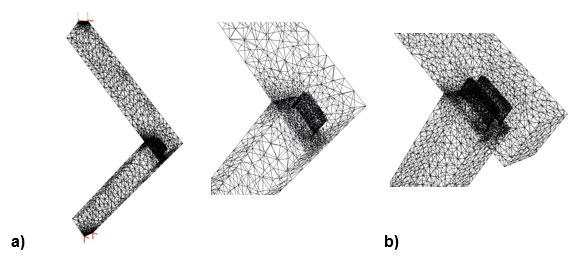Fig. 5. Numerical models of the joints: a) mesh of standard mortise and tenon joint with boundary conditions (tenon length 20 mm), b) details of standard and double mortise and tenon joints mesh (tenon length 20 mm)

Table 1. Elastic Properties of Beech Wood (Smardzewski 2008)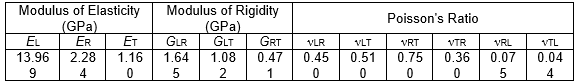RESULTS AND DISCUSSION

The experimental results of maximal (Fmax) and proportional force (Fp), maximal moment (Mmax) and proportional moment (Mp) and ratio of proportional and maximal moment (Mp /Mmax) of the standard tenon joints and double tenon joints with two different tenon lengths are given in Tables 2 and 3, respectively.

Generally, the results indicated that average bending moment (both maximum moment and proportional moment) of standard tenon joints and double tenon joints and for all investigated types of glue increased as the tenon length increased. Also, the results show relatively large differences among the average bending moment and the average proportional moments of mortise and tenon joints bonded by PVAc1, PVAc2, and PU. The differences are the results of a high slope of the proportional lines that have been fitted to the curves of force-displacement relationship in order to neglect the initial large displacements that occurred at the beginning of loading.

Table 2. Experimental Results of Forces and Bending Moments of the Standard Mortise and Tenon Joints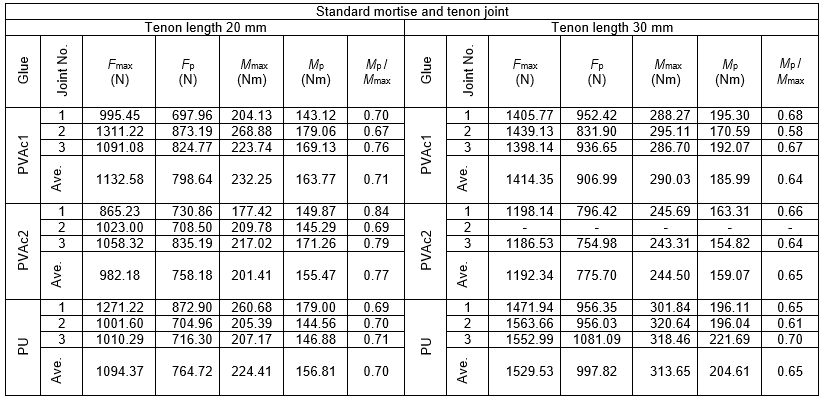Table 3. Experimental Results of Forces and Bending Moments of the Double Mortise and Tenon Joints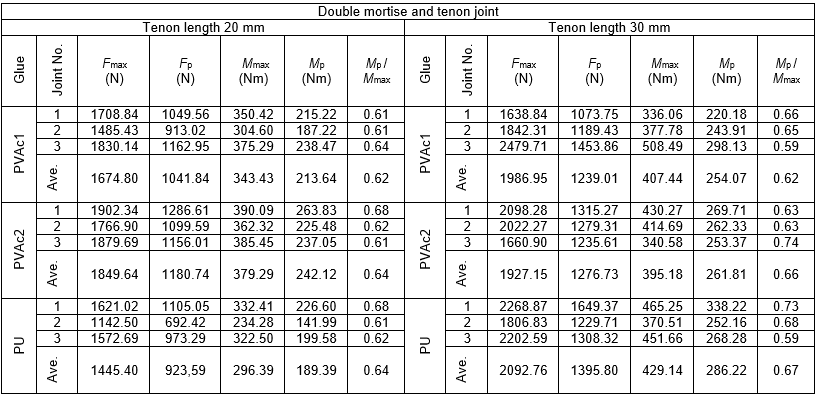The standard mortise and tenon joints glued with PVAc2 had the lowest average bending moment (201.4 Nm, 244.5 Nm) and average proportional moments (155.5 Nm, 159.1 Nm) for tenon length 20 mm and 30 mm, respectively. The joints glued with PVAc1 for tenon length 20 mm and the joints glued with PU for tenon length 30 mm had the highest average bending moment and proportional moment. The maximal bending moment (average value) increased by 24.9% (PVAc1), 21.4% (PVAc2) and 39.8% (PU) as tenon length increased from 20 mm to 30 mm for standard mortise and tenon joint. The joints with tenon length 30 mm had 13.6% (PVAc1), 2.3% (PVAc2) and 30.9% (PU) higher proportional bending moments (average value) than the joints with tenon length 20 mm. Normalized ratios of average values of proportional and maximal moment of the standard tenon joints with tenon length 20 mm were 71% (PVAc1), 77% (PVAc2) and 70% (PU). These percentages were lower for the standard tenon joints with tenon length 30 mm and those percentages are 64% (PVAc1), 65% (PVAc2) and 65% (PU).

The double mortise and tenon joints glued with PU had the lowest average bending moment (296.4 Nm) and average proportional moment (189.4 Nm) for tenon length 20 mm and also had the highest average bending moment (429.1 Nm) and average proportional moment (286.2 Nm) for tenon length 30 mm. The maximal bending moment of double mortise and tenon joints with tenon length 30 mm were 18.6% (PVAc1), 4.2% (PVAc2) and 44.8% (PU) higher than the average value for the double tenon joints with tenon length 20 mm. The average proportional moment increased by 18.9% (PVAc1), 8.1% (PVAc2) and 51.1% (PU) as tenon length increased from 20 mm to 30 mm for double mortise and tenon joint. The percentages of the average ratio of proportional and maximal moments of the double tenon joints with tenon length 20 mm were 62% (PVAc1), 64% (PVAc2) and 64% (PU), while they were 62% (PVAc1), 66% (PVAc2) and 67% (PU) for the double tenon joints with tenon length 30 mm.

The differences between the average value of maximum moment (bending moment capacities) of standard tenon joints and double tenon joints were clearly observed. However, these values were not comparable due to the dimensional differences of these two types of joints (thickness and width of tenon and thickness of joint elements). The presented experimental results are unable to determine the dimensional effect on the joints maximum bending moment. Also, the characteristic patterns of fractures of certain types of joints were not observed. Wood fracture of the joint member, tenon fracture or tenon pulled out from the member, as glue line failed and the tenon started to take the load, occurred in all sets of standard and double joints, as shown in Fig. 6.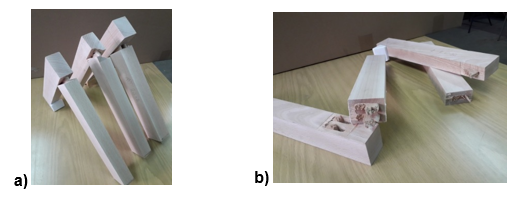Fig. 6. Type of standard and double joints failures: a) tenons pull out from the members after the glue line fractured and the fracture of wood of a joint member, b) the fracture of joints tenons

The analytical calculation results of the reaction moments, total reaction moment MR, and ratio of total reaction moment for tenon lengths 20 mm and tenon lengths 30 mm of the standard and double tenon joints are given in Table 4. The compression strength of beech wood normal to the fibers (negative normal stresses σ1 and σ2) and the generalized strength of a glue line (shear stress) that was used in the calculation was 10 MPa (Skarvelis and Mantanis 2013; Derikvand and Pangh 2016).

Table 4. Analytical Calculation of Reaction Moments of Standard and Double Tenon Joints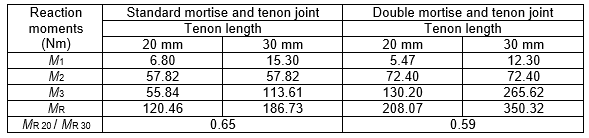Table 5. Ratio of Calculated Reaction Moments and Experimental Moments of Standard and Double Tenon Joints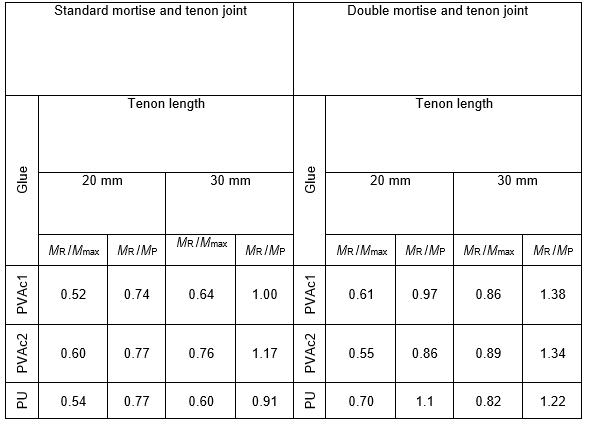The moment of the edge cheek M1 and the reactive moment in the plane of the face cheek M3 for tenon length 20 mm were 55.5% and 50.9% lower than the value for joints with tenon length 30 mm for both standard and double joints, respectively. Apparently, the value of the reaction moment of structural shoulder M2 did not change. The percentages of the ratio of total reaction moment for tenon lengths 20 mm and tenon lengths 30 mm were 65% and 59% for the standard tenon joints and for the double tenon joints, respectively.

The ratio of calculated total reaction moments and experimental moments for the standard and double tenon joints and tenon lengths 20 and 30 mm are given in Table 5. The results indicated that the reactive moment obtained by the analytical calculation does not represent the value of the bending moment capacities of joint. The reactive moment can be taken as the estimated value of the proportional moment of the loaded joint.

The total reaction moments were no higher than bending moment capacities. The percentages of the ratio of total reaction moments and average maximum moments (bending moment capacities) for tenon lengths 20 mm were ranged from 52% to 60% for the standard tenon joints and from 55% to 70% for the double tenon joints. The percentages of the same ratio for tenon lengths 30 mm were higher and ranged from 60% to 76% for the standard tenon joints and from 82% to 89% for the double tenon joints.

In general, the total reaction moments were lower than proportional moments for tenon length 20 mm or higher than proportional moments for tenon length 30 mm. The exception were some joints glued with PU. The percentages of the ratio of total reaction moments and proportional moments for tenon lengths 20 mm were ranged from 74% to 77% for the standard tenon joints and from 86% to 110% for the double tenon joints. The percentages of the same ratio for tenon lengths 30 mm were higher and were ranged from 91% to 117% for the standard tenon joints and from 122% to 138% for the double tenon joints.

Table 6. Experimental Results of Displacements and the Ratios of Forces and Displacements of the Standard Mortise and Tenon Joints